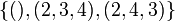# A3 in S5

This article is about a particular subgroup in a group, up to equivalence of subgroups (i.e., an isomorphism of groups that induces the corresponding isomorphism of subgroups). The subgroup is (up to isomorphism) cyclic group:Z3 and the group is (up to isomorphism) symmetric group:S5 (see subgroup structure of symmetric group:S5).
VIEW: Group-subgroup pairs with the same subgroup part | Group-subgroup pairs with the same group part | All pages on particular subgroups in groups

Let$G$ be the alternating group:A5, i.e., the alternating group (the group of all permutations) on the set$\{ 1,2,3,4,5 \}$.$G$ has order$5! = 120$.

Consider the subgroup:$H = H_{\{1,2,3 \}} = \{ (), (1,2,3), (1,3,2) \}$$H$ has a total of 10 conjugate subgroups (including$H$ itself) and the subgroups are parametrized by subsets of size 3 in$\{1,2,3,4,5 \}$ describing the support of the 3-cycles. The complementary subset of size two is fixed point-wise by that conjugate subgroup:

Subset of size 3 Complementary subset of size 2 fixed point-wise by the subgroup Conjugate of$H$
1,2,3 4,5$\! \{ (), (1,2,3), (1,3,2) \}$
1,2,4 3,5$\! \{ (), (1,2,4), (1,4,2) \}$
1,2,5 3,4$\! \{ (), (1,2,5), (1,5,2) \}$
1,3,4 2,5$\! \{ (), (1,3,4), (1,4,3) \}$
1,3,5 2,4$\! \{ (), (1,3,5), (1,5,3) \}$
1,4,5 2,3$\! \{ (), (1,4,5), (1,5,4) \}$
2,3,4 1,5$\! \{ (), (2,3,4), (2,4,3) \}$
2,3,5 1,4$\! \{ (), (2,3,5), (2,5,3) \}$
2,4,5 1,3$\! \{ (), (2,4,5), (2,5,4) \}$
3,4,5 1,2$\! \{ (), (3,4,5), (3,5,4) \}$

Each of these subgroups is isomorphic to cyclic group:Z3.

## Arithmetic functions

Function Value Explanation
order of the whole group 120$5! = 120$. See symmetric group:S5.
[[order of a group|order of the subgroup 3
index of the subgroup 40 Follows from Lagrange's theorem
size of conjugacy class of subgroups = index of normalizer 10
number of conjugacy classes in automorphism class 1

## Effect of subgroup operators

In the table below, we provide values specific to$H$.

Function Value as subgroup (descriptive) Value as subgroup (link) Value as group
normalizer$\langle (1,2,3), (1,2), (4,5) \rangle$ direct product of S3 and S2 in S5 dihedral group:D12
centralizer$\langle (1,2,3), (4,5) \rangle$ Z6 in S5 cyclic group:Z6
normal core trivial subgroup -- trivial group
normal closure the alternating subgroup A5 in S5 alternating group:A5
characteristic core trivial subgroup -- trivial group
characteristic closure the alternating subgroup A5 in S5 alternating group:A5

## Conjugacy class-defining functions

Conjugacy class-defining function What it means in general Why it takes this value
Sylow subgroup for the prime$p = 3$ A$p$-Sylow subgroup is a subgroup whose order is a power of$p$ and index is relatively prime to$p$. Sylow subgroups exist and Sylow implies order-conjugate, i.e., all$p$-Sylow subgroups are conjugate to each other. The order of this subgroup is 3, which is the largest power of 3 dividing the order of the group.